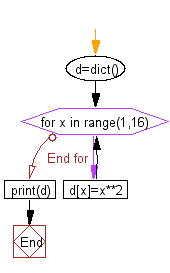﻿ Python: Print a dictionary where the keys are numbers between 1 and 15 and the values are square of keys - w3resource# Python: Print a dictionary where the keys are numbers between 1 and 15 and the values are square of keys

## Python dictionary: Exercise-7 with Solution

Write a Python script to print a dictionary where the keys are numbers between 1 and 15 (both included) and the values are square of keys.

Sample Solution:-

Python Code:

``````d=dict()
for x in range(1,16):
d[x]=x**2
print(d)
```
```

Sample Output:

```{1: 1, 2: 4, 3: 9, 4: 16, 5: 25, 6: 36, 7: 49, 8: 64, 9: 81, 10: 100, 11: 121, 12: 144, 13: 169, 14: 196, 15:
225}
```

Flowchart:## Visualize Python code execution:

The following tool visualize what the computer is doing step-by-step as it executes the said program:

Python Code Editor:

Have another way to solve this solution? Contribute your code (and comments) through Disqus.

What is the difficulty level of this exercise?

Test your Programming skills with w3resource's quiz.

﻿

## Python: Tips of the Day

Invokes the provided function after ms milliseconds:

Example:

```from time import sleep

def tips_delay(fn, ms, *args):
sleep(ms / 1000)
return fn(*args)
print(tips_delay(
lambda x: print(x),
1000,
'w3r'
))
```

Output:

```w3r
None
```